#Quality Digest

Featured Product
This Week in Quality Digest Live
Statistics Features
Donald J. Wheeler
What does this ratio tell us?
Harish Jose
Any statistical statement we make should reflect our lack of knowledge
Donald J. Wheeler
How to avoid some pitfalls
Kari Miller
CAPA systems require continuous management, effectiveness checks, and support
Donald J. Wheeler
What happens when the measurement increment gets too large?
Statistics News
How to use Minitab statistical functions to improve business processes
New capability delivers deeper productivity insights to help manufacturers meet labor challenges
Day and a half workshop to learn, retain, and transfer GD&T knowledge across an organization
Elsmar Cove is a leading forum for quality and standards compliance
InfinityQS’ quality solutions have helped cold food and beverage manufacturers around the world optimize quality and safety
User friendly graphical user interface makes the R-based statistical engine easily accessible to anyone
Collect measurements, visual defect information, simple Go/No-Go situations from any online device
Good quality is adding an average of 11 percent to organizations’ revenue growth
Ability to subscribe with single-user minimum, floating license, and no long-term commitment
Statistics

## ANOX: The Analysis of Individual Values

### A new test for homogeneity

Published: Tuesday, September 5, 2017 - 12:03Donald J. WheelerJames Beagle III

Sometimes we use a chart for individual values and a moving range (an XmR chart) to assess the homogeneity of a finite data set. Since this is an "off-label" use for the XmR chart, we first consider the drawbacks associated with using a sequential technique as a one-time test, and then present an adaptation of the X chart (the analysis of individual values or ANOX) that functions like other one-time statistical tests.

### The XmR chart

Like all process behavior charts, the XmR chart was designed for the sequential analysis of a continuing stream of observational data. Here the data will generally represent one condition, and the purpose of the chart is to identify unplanned changes in the underlying process. After the baseline period, where we compute the limits, we extend the limits forward and continue to add data to the chart. Each time we add an additional point to the chart we are performing an act of analysis. Each of these analyses asks if the current value is consistent with the baseline period. And, as with all sequential procedures, we want to perform each of these acts of analyses in a conservative manner in order to reduce the overall risk of a false alarm.

However, we do have to get started, and we do this with a baseline period. This choice of a baseline period is a matter of judgment. It has to make sense in the context of the application. Moreover, since future values will be compared to the baseline period, the choice of the baseline period will define the questions framed by the process behavior chart.

How many data are needed for the baseline period? While a rational baseline may end up using as few as four or five points, we prefer to have 17 to 30 points in our baseline when the context allows us to do so. On the high side, we will seldom need more than 50 points for a rational baseline on an XmR chart.

Once we have selected our baseline period and computed our three-sigma limits according to the usual formula  [ Average ± 2.66 (Average Moving Range) ], we are ready to begin the sequential analysis portion of the XmR chart. As we do this the inherently conservative nature of the three-sigma limits minimizes the risk of a false alarm for each individual act of analysis regardless of the shape of the histogram.

As an example consider some data from the classroom. A box containing 4,800 beads of different colors is sampled, with replacement, using a paddle with 50 holes. The paddle is dipped into the box, pulled out, and the number of yellow beads in the sample of 50 beads is recorded. After 20 such draws we have 20 counts, and these counts are used to create a baseline for future drawings from this bead box. The baseline portion of the XmR chart is shown on the left side of figure 1. It has an average of 5.15 and an average moving range of 3.26.Figure 1:
XmR chart for the number of yellow beads out of 50 beads

Next, as each additional drawing is made, the count of yellow beads is placed on the process behavior chart. As each point is added to the chart another act of analysis is performed. The question asked is whether the latest point is detectably different from the points in the baseline period? Twenty such sequential acts of analysis are shown on the right side of figure 1.

The fixed-width, three-sigma limits of the process behavior chart assure us that each of the sequential analyses is done in a conservative manner. This means that whenever we find an individual value outside the limits, we can be confident that that value is different from the values in the baseline period and a change is likely to have occurred in the underlying process. This time-ordered, sequential analysis is the essence of the process behavior chart technique.

### One-time tests

When we use a process behavior chart as a one-time test for a finite data set we are no longer characterizing the behavior a continuing process. We are simply using the front-end, baseline portion of the process behavior chart as a test of homogeneity for the data already in hand. This is a fundamental change in usage, with consequences that we will note below. Some examples of where this type of analysis would be in order are:

1. Characterization of a product in an engineering environment: A pilot run of products or assemblies may be produced to assess functional or design parameters. Since this pilot-run product may be used to determine specifications for components or for performance parameters, the homogeneity of the physical and functional characteristics of the pilot run will be of interest.

2. Evaluation of new designs in research runs: When a small number of items are evaluated for performance characteristics it is important to know that the items are homogeneous with regard to their design characteristics.

3. Representation: When a sample of items is obtained from a lot, our extrapolation from the sample back to the lot as a whole will depend upon how well the sample represents the lot. This representativeness will depend in part upon the homogeneity of the sample. If the sample is not homogeneous, then it is likely that the lot is also not homogenous. And if the lot is not homogeneous, no single sample can be said to be truly representative of the whole.

When we use an XmR chart as a one-time test it is usually inappropriate to use run tests. Run tests are built on the notion of sequence and patterns within that sequence, but with tests for homogeneity the sequence of the values will often be unknown or arbitrary. For this reason, unless we know and are using the time-order sequence for the data, we should only use points outside the limits as signals of a lack of homogeneity.

### Alpha levels for the XmR chart

When we use an XmR chart as a one-time test we are simply examining a fixed set of k values for evidence of a lack of homogeneity. With one-time tests it is customary to state the risk of a false alarm. (In the context of a test of homogeneity, a false alarm is equivalent to saying that a homogeneous data set is not homogeneous.)

The false alarm risk for the baseline portion of an XmR chart will be referred to as the "baseline alpha" level to distinguish it from the "alpha" level commonly cited for each individual test for the sequential portion of the XmR chart technique. The relation between these two quantities is given by Bonferroni's inequality. For a baseline containing k values, the baseline alpha will fall in between two values: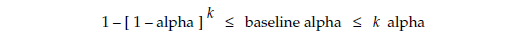If we use the traditional, normal-theory alpha value of 0.0027 for the individual tests in the sequential portion of an XmR chart, then a baseline consisting of k = 19 points would be said to have a baseline alpha of: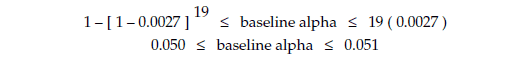Thus, when an XmR chart is used as a test for homogeneity for 19 values it can be said to have an overall risk of a false alarm of about 5 percent.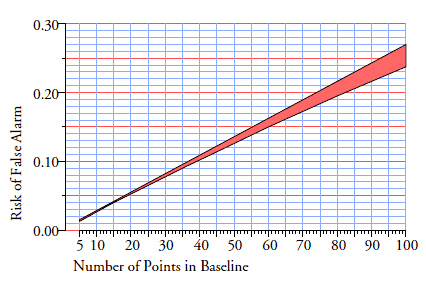Figure 2:
Overall alpha levels for XmR chart baselines with k values

When we plot the results of the Bonferroni inequality vs. the number of values in the baseline period of an XmR chart we get the graph shown in figure 2. When an XmR chart is used as a one-time test for the homogeneity of k values the likelihood of a false alarm will depend upon k. This means that, unlike other one-time tests, we do not get to choose our alpha level when we use an XmR chart as a test for homogeneity.

Typically with a one-time test we choose our alpha level, perform the test, state our conclusion, and live with the consequences. In some cases it will be more important to avoid false alarms, and in others it will be more important to avoid missed signals. By choosing a small alpha level (say 1%) we can minimize the risk of a false alarm. By choosing a large alpha level (say 10%) we can reduce the risk of a missed signal. Decision theory shows that for one-time tests the traditional alpha level of 5 percent strikes a reasonable balance between both mistakes. Thus, the choice of alpha level is how we show our attitude toward the analysis and fine-tune our one-time test.

So, the drawback to using an XmR chart as a test of homogeneity is the inability to choose our alpha level. Since the increasing risk of a false alarm shown in figure 2 is a consequence of the fixed-width limits used with the XmR chart, the obvious way to fix this problem is to use variable-width limits. This was the idea behind Ellis Ott's analysis of means (ANOM), and it is what we are proposing for the analysis of individual values (ANOX).

### The analysis of individual values (ANOX)

Given a set of k individual values and given the k–1 successive differences between these values (i.e., k–1 moving ranges), the homogeneity of this set of k individual values may be examined by computing the limits: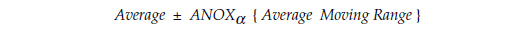Where the scaling factor ANOXα depends upon the desired alpha level for the test and the number of individual values being tested. When the k values are actually homogeneous these limits will bracket all of the individual values 100[1–alpha] percent of the time, and the smallest or largest of the k individual values can be expected to exceed these limits only 100[alpha] percent of the time.

If a single individual value falls outside the ANOX limits then you either have a rare event with probability alpha, or you have a nonhomogeneous set of individual values. Of course, as more values fall outside the ANOX limits, and as they fall further outside the ANOX limits, the evidence of nonhomogeneity becomes stronger.

In applying this test for homogeneity the individual values must not be arranged in a ranking (where the values are arranged in either an ascending or descending numerical order). Such orderings undermine the method of successive differences, which is the foundation of both the XmR chart and ANOX.

It is always best to use the data in the order in which they naturally occur. If time-order information is available, this ordering is preferred. If the time-order information has been lost, use the order in which the data are presented (as long as it is not a ranking).

### ANOX for blast furnace silicon

As an example, the data in figure 3 came from a print-out of 63 consecutive measurements of the silicon level in samples of hot metal coming from a blast furnace. The average is 149.9. Here we have no contextual information given along with the values, so we read the values in rows, compute the moving ranges, and find the average moving range to be 70.0.

Figure 11 shows the ANOX.10 scaling factor for k = 63 values to be 2.782. Thus we compute 10% ANOX limits of 149.9 ± 2.782 (70.0)  =  –44.0 to 344.8.  Since these data have a boundary value of zero, we report limits of 0 to 344.8, and no values fall outside these limits. The graph in figure 4 shows these values and their limits.Figure 3:  63 measurements of blast furnace silicon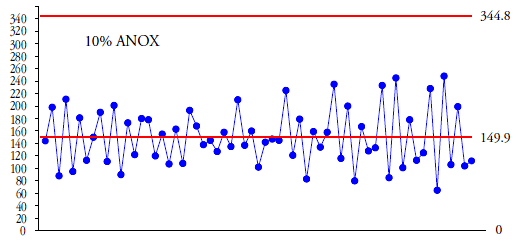Figure 4:
10% ANOX for blast furnace silicon

Anyone with much experience in looking at process behavior charts will be suspicious of the graph in figure 4. The running record is a sawtooth and the limits are exceedingly wide compared to the running record. So we might well decide to return to figure 3 and read the table in columns. When we do this we find that the average moving range drops to 15.9. Our revised 10% ANOX limits become 149.9 ± 2.782 (15.9)  =  105.7 to 194.2, and we get the graph in figure 5. Now we find 21 of the 63 values outside the 10% ANOX limits. Clearly these data are not homogeneous (and the silicon levels in the blast furnace are cycling over time).Figure 5:
Second 10% ANOX for blast furnace silicon

Here then is an illustration of the point that if any arbitrary ordering (other than a ranking) shows evidence of a lack of homogeneity, then the set of values should be considered to be nonhomogeneous. (If the data were truly homogeneous, every ordering would tell the same story.)

As soon as we determine that a data set is nonhomogeneous, all of the traditional statistical techniques that assume homogeneity for our data are off the table. They are no longer relevant. While the statistics will always describe the data set itself, a lack of homogeneity will undermine any attempt to interpret the statistics as representing anything outside the data set. (Our ability to extrapolate from a data set to a broader context will always depend upon the internal homogeneity of the data set.) With a lack of homogeneity the question immediately changes from "What do these data represent?" to "Why are these data not homogeneous?" Until we know the answer to the question of homogeneity we risk asking the wrong question of our data and taking the wrong action as a result.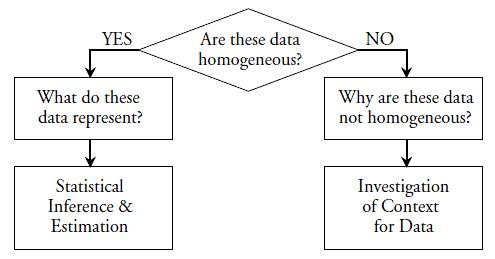Figure 6:
Asking the right question is essential to taking the right action

### Testing the sensors with ANOX

A set of planned experiments involved the use of sensors to detect the levels of specific carbohydrates in solution under various environmental conditions. Since the experimental results would depend upon multiple sensors giving equivalent results, a simple test was run prior to the experiment to evaluate the homogeneity of the collection of sensors available. First the 48 sensors were simultaneously exposed to a zero load (air) and the current in milliamps passing through each sensor was recorded. Next the sensors were simultaneously exposed to a high-end load using a known solution. Once again the current through each sensor was recorded.

The current readings for the zero load are shown in figure 7. Since it is very important that the sensors used in the experiment are all working the same, these values were tested for homogeneity at the 10% alpha level. (It was more important to find and eliminate any bad sensors than to erroneously delete some good sensors.)

The average is 0.8623 mA and the average moving range is 0.3051 mA. With k = 48 the ANOX.10 scaling factor is 2.706, giving 10% ANOX limits of 0.037 and 1.688.Figure 7:  Sensor currents under zero-load condition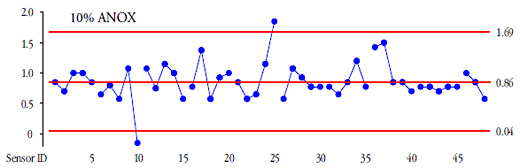Figure 8:  10% ANOX for sensor currents under zero load

Clearly sensors 10 and 25 are different from the rest under the zero-load condition. These two sensors should not be used in the upcoming experiments.

The current readings for the high-load condition are shown in figure 9. The average is 9.896 mA and the average moving range is 0.7689 mA. With k = 48 the ANOX.10 scaling factor is 2.706, giving 10% ANOX limits of 7.82 and 11.98.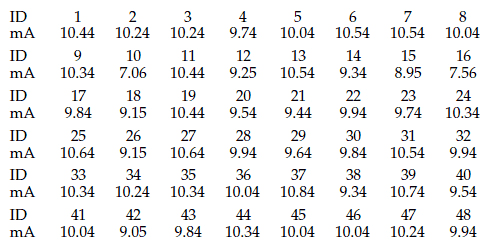Figure 9:
Sensor currents under high load condition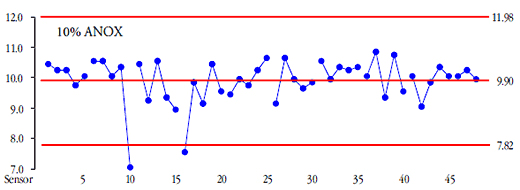Figure 10:  10% ANOX for sensor currents under high load

Here we find sensors 10 and 16 to be different from the rest under the high-load condition. So, we add sensor 16 to the "do not use" list. By using 10% ANOXs to test for homogeneity we can be confident that the remaining 45 sensors give similar readings under both zero-load and high-load conditions.

### The choice of alpha level

In most one-time tests we are trying to establish that a signal exists. In a test of homogeneity we are trying to establish that a signal does not exist. This difference will affect the way we use a test for homogeneity.

When we use a 5% ANOX or a 10% ANOX and find no evidence of a lack of homogeneity, then we can be comfortable with the conclusion that the data are probably homogeneous. We will have obtained a fairly strong result.

When we use a 1% ANOX and find evidence of a lack of homogeneity, then we can be comfortable with the conclusion that the data are not homogeneous.

Since the skeptic will assume the data are not homogeneous until proven otherwise, and since the strong conclusion regarding homogeneity requires a larger alpha level, we should be careful about using a 1% ANOX to conclude that the data are homogeneous. In the preceding examples, sensor 10 is outside the 1% ANOX limits in both cases, but sensors 25 and 16 are not. Would you want to risk using sensors 25 and 16 in your experiments just because they did not exceed the 1% ANOX limits?

In addition, it is important to remember that with ANOX the alpha level is the probability that either the maximum or minimum of the k points will fall outside the limits. So if we perform a 10% ANOX with k = 100 points, the alpha level of 10 percent does not mean that we expect 10 out of a 100 values to fall outside the limits. Instead, it means that there is a 10-percent chance that either the smallest or the largest of the 100 values might fall just outside the limits. When a false alarm occurs with ANOX it will tend to show a single point just outside the limit, rather than having a point noticeably outside the limits. The judgment that a data set is not homogeneous can depend upon how far outside the limits a point may fall as well as how many points are outside the limits.

### Comparing ANOX with the XmR chart

Since the whole of statistical inference is based on the assumption of homogeneity, it is appropriate to begin any analysis with a test of homogeneity.

From the Bonferroni inequality and figure 2 we may conclude that when we have fewer than 38 data, using an XmR chart as a one-time test will detect fewer signals of nonhomogeneity than will a 10% ANOX.

When we have fewer than 19 data, using an XmR chart as a one-time test will detect fewer signals of nonhomogeneity than will a 5% ANOX.

However, when we have 8 or more data, using a 1% ANOX will detect fewer signals of nonhomogeneity than will be found using an XmR chart as a one-time test.

Thus, ANOX is recommended to avoid the overly large alpha levels that automatically occur when we use an XmR chart as a one-time test with large data sets. Like virtually all other statistical procedures, ANOX provides you with a one-time test for homogeneity that has a user-selected risk of a false alarm.  However, unlike other statistical procedures, ANOX is not built upon the assumption that the data are homogeneous.

### Appendix One: Creating the ANOX Tables

Given a set of k individual values:denote the largest and smallest of these values by:Then the greatest deviation from the Average would be given by: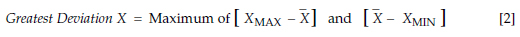If we let GDX90 denote the 90th percentile of the distribution of the Greatest Deviation Statistic, and if we define:then the quantity ANOX.10 will be defined by the 90th percentile of the distribution of the ratio: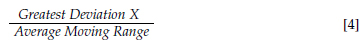where the average moving range statistic in the denominator is the average of the k–1 successive differences between the individual values.

In a similar manner the quantities ANOX.05 and ANOX.01 will be defined by the 95th and 99th percentiles of the distribution of the ratio in equation  above. To estimate these per­centiles for different values of k each of the authors independently ran a series of simulation runs. These independent simulations converged on the values given in the tables. (When corresponding results of the independent simulations were tested for detectable differences over 98 percent of the tests found no detectable difference at the five-percent level. At the one-percent level there were no detectable differences between the two independent simulations. Thus each author provided nontrivial confirmation for the other's results.)

A simulation run would begin with the generation of 10,000 random samples of size k using a standard normal distribution. For each of these samples the moving ranges were computed and the ratio in equation  above was computed. The 99th, 95th, and 90th per­centiles of the distribution of the ratio above would be estimated by finding the 100th, 500th, and 1000th values from the ordered set of observed ratios. Thus, for each value of k, a simulation run would yield a single estimate for ANOX.01, a single estimate for ANOX.05, and a single estimate for ANOX.10. By re­peating this whole process dozens to hundreds of times for each value of k, and then averaging the resulting estimates, it was possible to obtain esti­mates for ANOX.01, ANOX.05, and ANOX.10 having very small uncertainties.

Since scaling factors such as these should form a smooth curve when plotted as a function of k, the estimates from the simulation studies were plotted along with their error bars, and the resulting curves were smoothed by adjusting points that seemed high or low relative to adjacent estimates. All but four of the adjusted values remained within the error bars from the simulation study, and these four were only 0.001 or 0.002 outside their error bars. Thus, the smoothed values given in the table form internally consistent sets that are also completely consistent with the simulation studies.

ANOX scaling factors for fewer than eight data are not given because of a quirk of the method of successive differences. For both an XmR chart and ANOX, when k is less than 8, it is only the first or last points that can fall outside the computed limits. This means that ANOX cannot provide a fair test of homogeneity for all the points in a data set until k is eight or greater.

In figure 11, the ANOX.10 values for k less than 170 all have a probable error of 0.001 or less. These values err by one unit or less in the third decimal place at least half the time. Hence they are essentially known to three decimal places. The ANOX.10 values for k greater than 170 have a probable error of 0.002 or less.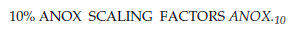For limits having a 10-percent chance of exceedence for max or min of k values.
Use when skeptical about homogeneity of the individual values.Figure 11:  10% ANOX scaling factors

In figure 12, the ANOX.05 values for k less than 30 have a probable error of 0.001. The remainder of the ANOX.05 values have a probable error of 0.002. This means that all the values in figure 12 err by two units or less in the third decimal place at least half the time. Hence, these values are still effectively known to three decimal places.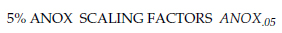For limits having a 5-percent chance of exceedence for max or min of k values.
Use when neutral about assumption of homogeneity for the individual values.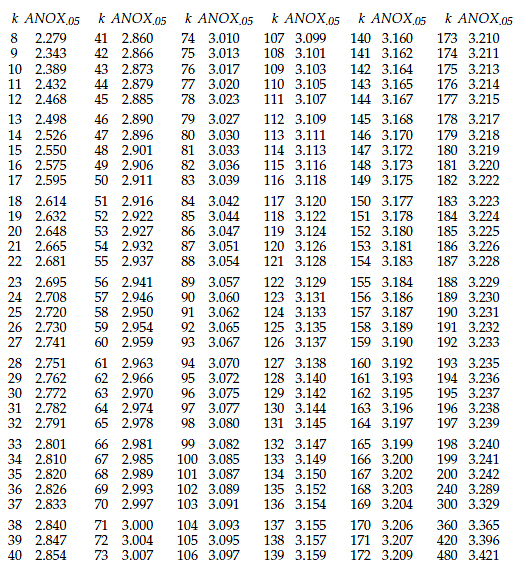Figure 12:  5% ANOX scaling factors

In figure 13, the ANOX.01, values for k less than 44 have a probable error of 0.002. For k greater than 44 they have a probable error of 0.003 or 0.004. So, while these values have some softness in the third decimal place, this softness is still smaller than the uncertainty introduced by rounding these values off to two decimal places. Therefore, these values are listed with three decimal places, even though the third decimal places are somewhat uncertain.For limits having a 1-percent chance of exceedence for max or min of k values.
Use only when gullible about assumption of homogeneity for individual values.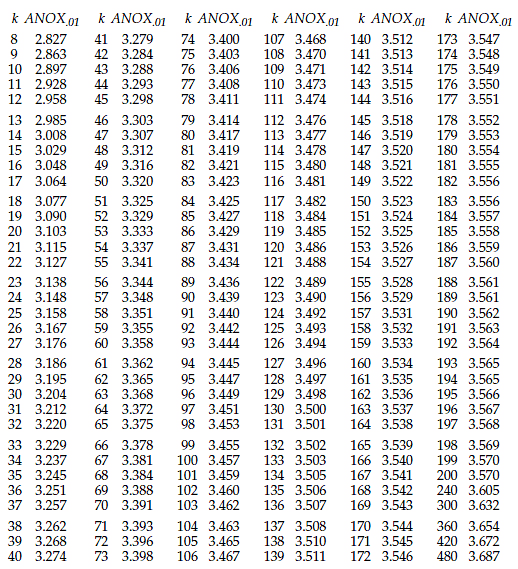Figure 13:  1% ANOX scaling factors

### Appendix Two: Programming the ANOX Tables

For programming purposes, when the number of values, k, is between 18 and 360, two-decimal place approximations for the ANOX scaling factors in figures 11, 12, and 13 may be obtained using equations of the following form with the coefficients in figure 14.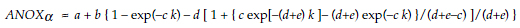Figure 14:  Coefficients for programming the ANOX tables

The use of these equations to estimate the ANOX scaling factors will result in limits that will have approximately the stated risk of a false alarm. These equations are not suitable for k < 18, or for k > 360, because they give values that differ from the tabled values by two or more units in the second decimal place. Thus, while a table lookup will be needed for k = 8 to 17, the equations above can be used to approximate the ANOX limits for k up to 360.

In practice, it will be a rare event for a data set consisting of several hundred values to be found to be homogeneous. Remember the ANOX scaling factors define limits that will be exceeded by the maximum or minimum value either 1, 5, or 10 percent of the time. As more values fall outside the ANOX limits, and as these values fall further outside the limits, the evidence of a lack of homogeneity grows ever stronger.

### Discuss### Donald J. Wheeler

Dr. Wheeler is a fellow of both the American Statistical Association and the American Society for Quality who has taught more than 1,000 seminars in 17 countries on six continents. He welcomes your questions; you can contact him at djwheeler@spcpress.com.### James Beagle III

James Beagle III is a lapsed CQE, CRE, and C6SBB (asking the wrong questions and obsessing about impractical concepts, before realizing what is important) with 35+years experience in process, quality, and reliability engineering. He primarily works with a large manufacturing base supplying data storage component products, where he develops tools for data analysis, designs experiments for product improvements, and works on product and process qualification. In his free time he works on numerical modeling (and is also known to have guitars at home). He can be reached at james@idealstates.net.